Courses

# Test: Introduction To Matrices

## 10 Questions MCQ Test Mathematics (Maths) Class 12 | Test: Introduction To Matrices

Description
This mock test of Test: Introduction To Matrices for JEE helps you for every JEE entrance exam. This contains 10 Multiple Choice Questions for JEE Test: Introduction To Matrices (mcq) to study with solutions a complete question bank. The solved questions answers in this Test: Introduction To Matrices quiz give you a good mix of easy questions and tough questions. JEE students definitely take this Test: Introduction To Matrices exercise for a better result in the exam. You can find other Test: Introduction To Matrices extra questions, long questions & short questions for JEE on EduRev as well by searching above.
QUESTION: 1

###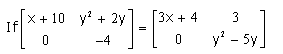Then the value of x is ____

Solution: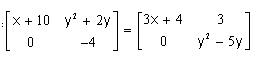= x+10 = 3x+4
= x = 3

QUESTION: 2

###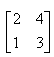is a matrix of order

Solution:

Since, there are 2 rows and 2 columns in the matrix, order of the matrix is 2*2.

QUESTION: 3

### For what real value of y will matrix A be equal to matrix B, where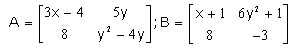​

Solution:

⇒ y2 - 4y = -3

⇒ y2 - 4y + 3 = 0

⇒ y2 -3y - y +3 = 0

⇒ y (y - 3) -1 (y-3) = 0

⇒(y - 1) (y - 3) = 0

⇒ y = 1,3

But there is not such option

We have another equation:

⇒ 5y = 6y2 + 1

⇒ 6y2 -5y +1 = 0

⇒ 6y2 -3y - 2y + 1 = 0

⇒ 3y (2y - 1) - 1 (2y - 1) = 0

⇒ (3y - 1) (2y - 1) = 0

⇒ y = 1/2, 1/3

Hence value of y is 1/2, 1/3.

QUESTION: 4

The number of all the possible matrices of order 2 × 2 with each entry 0, 1 or 2 is

Solution:

The number of elements in a 2 x 2 matrix is the product 2 x 2 =4

Each element can either be a 0,1 or 2.

Given this, the total possible matrices that can be selected is 34

32x2= 34= 81

QUESTION: 5

Consider the following information regarding the number of men and women workers in three BPOs I, II and III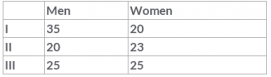What does the entry in the second row and first column represent if the information is represented as a 3 x 2 matrix?​

Solution:
QUESTION: 6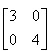is example of

Solution:

A matrix having non-zero elements only in the diagonal running from the upper left to the lower right.

The given matrix is a diagonal matrix.

QUESTION: 7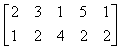is a matrix of order

Solution:

There are 2 rows and 5 columns in the matrix.
Therefore, order of the matrix: 2 x 5.

QUESTION: 8

What is the element in the 2nd row and 1st column of a 2 x 2 Matrix A = [ aij], such that a = (i + 3) (j – 1)​?

Solution:

Rows are represented by i
Columns are represented by j
... a=(i+3)(j-1)
i=2 , j=1    (given)
a21 = (2+3)(1-1)=0
so, a=0

QUESTION: 9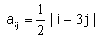the value of a22 is​

Solution:

aij = 1/2 |i-3j|
As aij = a22 ie i = 2 and j = 2
By substituting the values in the equation, we get
a22 = 1/2 |2-3(2)| = 1/2 |-4| = 2

QUESTION: 10

To construct a 2 x 3 matrix [ aij], such that aij = –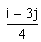The values that i and j can take are …….

Solution: